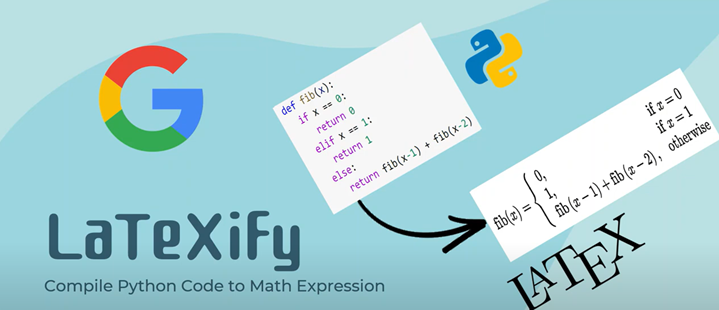# Scikit-Learn学习之交叉验证

## 留出法（Holdout cross validation）

• 简单易用，计算量较小。
• 对于大规模数据集，可以快速构建并验证模型。

• 训练集和测试集的划分可能不够随机或不够充分，导致结果偏差。
• 只进行一次划分，对于数据量较小时可能无法保证模型稳定性。
• 数据集划分后会浪费部分数据，尤其是当数据集非常小的时候。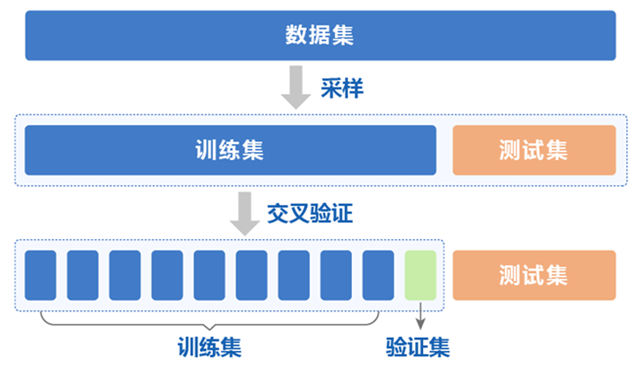• 训练集：训练模型
• 验证集：调参
• 测试集：评估模型的泛化能力

Scikit-Learn中的数据集拆分train_test_split()方法可以用于留出法。由于先前已经介绍过这里就不再做重复介绍了。

## k 折交叉验证（k-fold cross validation）

k折交叉验证首先将原始数据随机分成k个互斥的子集，然后利用其中的k-1个子集作为训练集，剩下的1个子集作为验证集。对于这k种划分情况，分别进行k次训练和测试，最终返回平均测试结果。

• 数据集的每个子集都有机会作为验证集和训练集，增加了模型的稳定性和精度。
• 能够更好地利用数据，相对于留出法和留一法更加可靠。

• 计算量相对于留出法和留一法略大。
• 在某些特殊情况（如数据分布不均衡、数据中存在噪声等）下，结果可能不太可靠。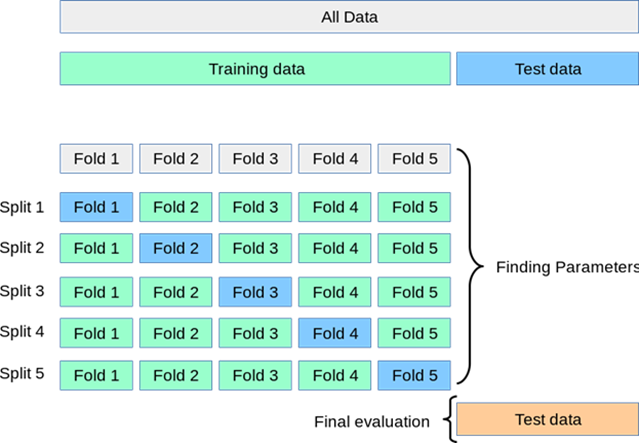### Scikit-learn中的k 折交叉验证

KFold、cross_val_score和cross_validate都是Scikit-learn中用于K折交叉验证的工具，它们的主要区别在于使用方式、返回结果和功能。

• 使用方式：
• KFold是一个交叉验证器，用于生成训练/测试集的索引，让我们可以采用自己的算法进行交叉验证。KFold提供了比较灵活的交叉验证功能，可以通过设置参数来控制分割数据集的方式，并可以将其与任何Scikit-learn估计器结合使用。
• cross_val_score是一种更为高级的接口，它封装了交叉验证中重复和平均化得分的过程，同时也可以和任何Scikit-learn估计器结合使用。因此，我们可以非常方便地进行模型评估、选择和调参。
• cross_validate相对于cross_val_score更加灵活，可以支持多种评价指标，包括准确率、F1值、精确率、召回率等，并且可以通过设置return_estimator参数来返回评估过程中生成的全部模型实例，方便进一步分析和调整模型。
• 返回结果：
• KFold只返回交叉验证的划分结果，不进行评估得分。
• cross_val_score函数仅返回交叉验证的得分。cross_validate函数除了交叉验证得分外，还可返回每个测试集上的训练时间和预测时间等信息，这对于模型评估和性能分析更加有帮助。
• 功能：
• KFold提供了比较灵活的交叉验证功能，可以通过设置参数来控制分割数据集的方式，并可以将其与任何Scikit-learn估计器结合使用。
• cross_val_score函数相对于KFold更为简单，只需传入分类器、特征矩阵X、标签y、交叉验证折数cv等参数即可。而cross_validate的使用方式则多了一些参数，如待评估的指标 scoring、是否返回拟合时间 return_train_score 等。
• cross_validate相较于cross_val_score函数更为灵活，可以支持多种评价指标，包括准确率、F1值、精确率、召回率等，并且可以通过设置return_estimator参数来返回评估过程中生成的全部模型实例，方便进一步分析和调整模型。

KFold的使用

from sklearn.datasets import load_iris
from sklearn.model_selection import KFold
from sklearn.linear_model import LogisticRegression

# 加载鸢尾花数据集
X, y = iris.data, iris.target

# 定义5折交叉验证器
kf = KFold(n_splits=5, shuffle=True, random_state=42)

# 创建逻辑回归模型
lr = LogisticRegression()

# 对于每个训练集和测试集进行训练和评估
for train_index, test_index in kf.split(X):
X_train, X_test = X[train_index], X[test_index]
y_train, y_test = y[train_index], y[test_index]

# 训练模型
lr.fit(X_train, y_train)

# 评估模型
score = lr.score(X_test, y_test)
print("Accuracy:", score)


cross_val_score的使用

from sklearn.datasets import load_iris
from sklearn.model_selection import cross_val_score
from sklearn.linear_model import LogisticRegression

# 加载鸢尾花数据集
X, y = iris.data, iris.target

# 创建逻辑回归模型
lr = LogisticRegression()

# 进行5折交叉验证
scores = cross_val_score(lr, X, y, cv=5, scoring="accuracy")

# 输出所有测试集得分的平均值和标准差
print("Accuracy: %0.2f (+/- %0.2f)" % (scores.mean(), scores.std() * 2))


cross_validate的使用

rom sklearn.datasets import load_iris
from sklearn.model_selection import cross_validate
from sklearn.linear_model import LogisticRegression

# 加载鸢尾花数据集
X, y = iris.data, iris.target

# 创建逻辑回归模型
lr = LogisticRegression()

# 进行5折交叉验证，计算准确率和训练时间
scoring = ["accuracy", "neg_mean_squared_error"]
results = cross_validate(lr, X, y, cv=5, scoring=scoring, return_train_score=True)

# 输出所有测试集得分的平均值和标准差
print("Test accuracy: %0.2f (+/- %0.2f)" % (results["test_accuracy"].mean(), results["test_accuracy"].std() * 2))

# 输出所有训练集得分的平均值和标准差
print("Train accuracy: %0.2f (+/- %0.2f)" % (results["train_accuracy"].mean(), results["train_accuracy"].std() * 2))

# 输出拟合时间和评估时间的平均值和标准差
print("Fit time: %0.2f (+/- %0.2f)" % (results["fit_time"].mean(), results["fit_time"].std() * 2))
print("Score time: %0.2f (+/- %0.2f)" % (results["score_time"].mean(), results["score_time"].std() * 2))


### GroupKFold、RepeatedKFold、StratifiedKFold和RepeatedStratifiedKFold

GroupKFold

GroupKFold是一种交叉验证的策略，它将数据集按照分组信息进行划分，保证同一组中的样本要么全部在训练集中，要么全部在测试集中。

GroupKFold的主要参数包括：

• n_splits：表示将数据集拆分成几份，默认为5。

GroupKFold的优点是能够确保同一组中的样本要么全部在训练集中，要么全部在测试集中，从而更好地反映模型的实际表现。缺点是对于某些数据集和模型，可能需要更多的训练时间和计算资源。

from sklearn.datasets import make_classification
from sklearn.model_selection import GroupKFold
from sklearn.linear_model import LogisticRegression
from sklearn.metrics import accuracy_score

# 生成分类数据集和分组信息
X, y = make_classification(n_samples=1000, n_features=10, random_state=42)
groups = [i % 3 for i in range(len(X))]

# 定义GroupKFold对象
gkf = GroupKFold(n_splits=3)

# 定义模型
model = LogisticRegression()

# 用GroupKFold进行交叉验证
scores = []
for train_index, test_index in gkf.split(X, y, groups):
X_train, X_test = X[train_index], X[test_index]
y_train, y_test = y[train_index], y[test_index]
model.fit(X_train, y_train)
y_pred = model.predict(X_test)
score = accuracy_score(y_test, y_pred)
scores.append(score)

# 输出结果
print("Accuracy: {:.3f} (+/- {:.3f})".format(np.mean(scores), np.std(scores)))


RepeatedKFold

RepeatedKFold是一种交叉验证的策略，它将数据集随机拆分为训练集和测试集，可以用于评估模型的性能和选择最优参数。与KFold不同的是，RepeatedKFold会重复运行K次，每次生成不同的随机拆分结果。

RepeatedKFold的主要参数包括：

• n_splits：表示将数据集拆分成几份，默认为5。
• n_repeats：表示重复运行几次交叉验证，默认为10。

RepeatedKFold的优点是能够生成多个随机拆分结果，从而减小误差和提高可靠性。缺点是由于每次随机拆分都需要重新生成，因此计算代价较大，适合数据量较小的场景。

from sklearn.datasets import load_iris
from sklearn.model_selection import RepeatedKFold
from sklearn.tree import DecisionTreeClassifier
from sklearn.metrics import accuracy_score

# 加载鸢尾花数据集

# 定义RepeatedKFold对象
rkf = RepeatedKFold(n_splits=5, n_repeats=3, random_state=42)

# 定义模型
model = DecisionTreeClassifier()

# 用RepeatedKFold进行交叉验证
scores = []
for train_index, test_index in rkf.split(X):
X_train, X_test = X[train_index], X[test_index]
y_train, y_test = y[train_index], y[test_index]
model.fit(X_train, y_train)
y_pred = model.predict(X_test)
score = accuracy_score(y_test, y_pred)
scores.append(score)

# 输出结果
print("Accuracy: {:.3f} (+/- {:.3f})".format(np.mean(scores), np.std(scores)))


StratifiedKFold

StratifiedKFold是一种交叉验证的策略，它将数据集按照类别信息进行分层划分，可以确保每个类别在训练集和测试集中的比例相同。

StratifiedKFold的主要参数包括：

• n_splits：表示将数据集拆分成几份，默认为5。

StratifiedKFold的优点是能够确保每个类别在训练集和测试集中的比例相同，从而更好地反映模型的实际表现。缺点是对于某些数据集和模型，可能需要更多的训练时间和计算资源。

from sklearn.datasets import load_iris
from sklearn.model_selection import StratifiedKFold
from sklearn.tree import DecisionTreeClassifier
from sklearn.metrics import accuracy_score

# 加载鸢尾花数据集

# 定义StratifiedKFold对象
skf = StratifiedKFold(n_splits=5)

# 定义模型
model = DecisionTreeClassifier()

# 用StratifiedKFold进行交叉验证
scores = []
for train_index, test_index in skf.split(X, y):
X_train, X_test = X[train_index], X[test_index]
y_train, y_test = y[train_index], y[test_index]
model.fit(X_train, y_train)
y_pred = model.predict(X_test)
score = accuracy_score(y_test, y_pred)
scores.append(score)

# 输出结果
print("Accuracy: {:.3f} (+/- {:.3f})".format(np.mean(scores), np.std(scores)))


RepeatedStratifiedKFold

RepeatedStratifiedKFold是一种交叉验证的策略，它将数据集按照类别信息进行分层划分，可以确保每个类别在训练集和测试集中的比例相同。与StratifiedKFold不同的是，RepeatedStratifiedKFold会重复运行K次，每次生成不同的随机拆分结果。

RepeatedStratifiedKFold的主要参数包括：

• n_splits：表示将数据集拆分成几份，默认为5。
• n_repeats：表示重复运行几次交叉验证，默认为10。

RepeatedStratifiedKFold的优点是能够确保每个类别在训练集和测试集中的比例相同，从而更好地反映模型的实际表现。缺点是由于每次随机拆分都需要重新生成，因此计算代价较大，适合数据量较小的场景。

from sklearn.datasets import load_digits
from sklearn.model_selection import RepeatedStratifiedKFold
from sklearn.linear_model import LogisticRegression
from sklearn.metrics import accuracy_score

# 加载手写数字数据集

# 定义RepeatedStratifiedKFold对象
rskf = RepeatedStratifiedKFold(n_splits=5, n_repeats=3, random_state=42)

# 定义模型
model = LogisticRegression()

# 用RepeatedStratifiedKFold进行交叉验证
scores = []
for train_index, test_index in rskf.split(X, y):
X_train, X_test = X[train_index], X[test_index]
y_train, y_test = y[train_index], y[test_index]
model.fit(X_train, y_train)
y_pred = model.predict(X_test)
score = accuracy_score(y_test, y_pred)
scores.append(score)

# 输出结果
print("Accuracy: {:.3f} (+/- {:.3f})".format(np.mean(scores), np.std(scores)))


## 留一法（Leave one out cross validation）

• 将原始数据集的全部信息都用于训练模型，从而得到最准确的模型评估结果。
• 对于小数据集比较适用。

• 运算开销较大，需要训练n个模型，其中n为数据集大小。
• 对于大数据集，由于需要进行大量的重复实验，留一法计算成本很高。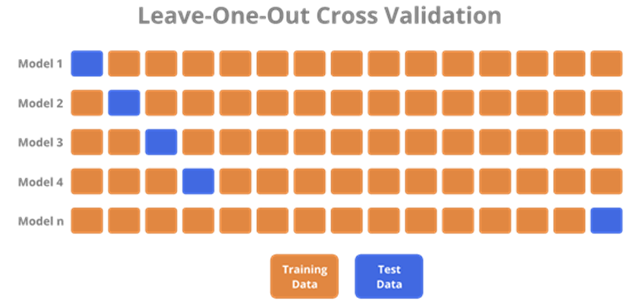Scikit-learn中可以使用LeaveOneOut类来进行留一法交叉验证（Leave-One-Out Cross Validation）。留一法交叉验证是将每个样本都作为测试数据，其余样本作为训练数据，这样得到n个分类器的评估结果，最后计算平均值。对于小规模数据集（如当样本数量小于20时），留一法交叉验证是一种很好的验证方法。

from sklearn.datasets import load_iris
from sklearn.model_selection import LeaveOneOut
from sklearn.linear_model import LogisticRegression

# 加载鸢尾花数据集
X, y = iris.data, iris.target

# 创建逻辑回归模型
lr = LogisticRegression()

# 定义留一法交叉验证器
loo = LeaveOneOut()

# 初始化正确分类数和总样本数
correct = 0
total = 0

# 对于每个训练集和测试集进行训练和评估
for train_index, test_index in loo.split(X):
X_train, X_test = X[train_index], X[test_index]
y_train, y_test = y[train_index], y[test_index]

# 训练模型
lr.fit(X_train, y_train)

# 预测测试集并计算准确率
y_pred = lr.predict(X_test)
if y_pred == y_test:
correct += 1
total += 1

# 输出模型在所有测试集上的准确率
print("Accuracy:", correct / total)


## 留P法（Leave-P-Out，LPO）交叉验证

Scikit-learn中可以使用LeavePOut类来实现LPO交叉验证。下面是一个使用留P法交叉验证进行模型评估的示例：

from sklearn.datasets import load_iris
from sklearn.model_selection import LeavePOut
from sklearn.linear_model import LogisticRegression

# 加载鸢尾花数据集
X, y = iris.data, iris.target

# 创建逻辑回归模型
lr = LogisticRegression()

# 定义留P法交叉验证器
lpo = LeavePOut(p=2)

# 初始化正确分类数和总样本数
correct = 0
total = 0

# 对于每个训练集和测试集进行训练和评估
for train_index, test_index in lpo.split(X):
X_train, X_test = X[train_index], X[test_index]
y_train, y_test = y[train_index], y[test_index]

# 训练模型
lr.fit(X_train, y_train)

# 预测测试集并计算准确率
y_pred = lr.predict(X_test)
if y_pred == y_test:
correct += 1
total += 1

# 输出模型在所有测试集上的准确率
print("Accuracy:", correct / total)


## 随机排列交叉验证ShuffleSplit

ShuffleSplit是一种交叉验证的策略，它将数据随机拆分为训练集和测试集，可以用于评估模型的性能和选择最优参数。

ShuffleSplit的主要参数包括：

• n_splits：表示拆分成几份，默认为10。
• test_size：表示每次拆分中测试集的大小，可以是一个浮点数（表示占总数据量的比例）或整数（表示具体数量），默认为1。
• train_size：表示每次拆分中训练集的大小，如果不设置，则默认为1-test_size。
• random_state：表示随机数种子，用于确保每次拆分结果的可重复性。

ShuffleSplit的优点是可以利用全部数据对模型进行训练和测试，并且每次划分的结果都是随机的，能够更好地反映模型的实际表现。缺点是由于每次随机划分都需要重新生成，因此计算代价较大，适合数据量较小的场景。

from sklearn.datasets import load_digits
from sklearn.model_selection import ShuffleSplit
from sklearn.linear_model import LogisticRegression
from sklearn.metrics import accuracy_score

# 加载手写数字数据集

# 定义ShuffleSplit对象
ss = ShuffleSplit(n_splits=5, test_size=0.2, random_state=42)

# 定义模型
model = LogisticRegression()

# 用ShuffleSplit进行交叉验证
scores = []
for train_index, test_index in ss.split(X):
X_train, X_test = X[train_index], X[test_index]
y_train, y_test = y[train_index], y[test_index]
model.fit(X_train, y_train)
y_pred = model.predict(X_test)
score = accuracy_score(y_test, y_pred)
scores.append(score)

# 输出结果
print("Accuracy: {:.3f} (+/- {:.3f})".format(np.mean(scores), np.std(scores)))


## 分层K折交叉验证StratifiedKFold

StratifiedKFold的主要参数包括：

• n_splits：表示将数据集拆分成几份，默认为5。
• shuffle：表示是否对数据集进行随机打乱，默认为True。
• random_state：表示随机数种子，用于确保每次拆分结果的可重复性。

StratifiedKFold的优点是能够确保不同类别的样本在训练集和测试集中的比例相同，从而更好地反映模型的实际表现。缺点是对于某些数据集和模型，可能需要更多的训练时间和计算资源。

from sklearn.datasets import load_digits
from sklearn.model_selection import StratifiedKFold
from sklearn.linear_model import LogisticRegression
from sklearn.metrics import accuracy_score

# 加载手写数字数据集

# 定义StratifiedKFold对象
skf = StratifiedKFold(n_splits=5, shuffle=True, random_state=42)

# 定义模型
model = LogisticRegression()

# 用StratifiedKFold进行交叉验证
scores = []
for train_index, test_index in skf.split(X, y):
X_train, X_test = X[train_index], X[test_index]
y_train, y_test = y[train_index], y[test_index]
model.fit(X_train, y_train)
y_pred = model.predict(X_test)
score = accuracy_score(y_test, y_pred)
scores.append(score)

# 输出结果
print("Accuracy: {:.3f} (+/- {:.3f})".format(np.mean(scores), np.std(scores)))


## 分层随机排列交叉验证StratifiedShuffleSplit

StratifiedShuffleSplit的主要参数包括：

• n_splits：表示拆分成几份，默认为10。
• test_size：表示每次拆分中测试集的大小，可以是一个浮点数（表示占总数据量的比例）或整数（表示具体数量），默认为1。
• train_size：表示每次拆分中训练集的大小，如果不设置，则默认为1-test_size。
• random_state：表示随机数种子，用于确保每次拆分结果的可重复性。

StratifiedShuffleSplit的优点是能够确保不同类别的样本在训练集和测试集中的比例相同，从而更好地反映模型的实际表现。缺点是由于每次随机划分都需要重新生成，因此计算代价较大，适合数据量较小的场景。

from sklearn.datasets import load_digits
from sklearn.model_selection import StratifiedShuffleSplit
from sklearn.linear_model import LogisticRegression
from sklearn.metrics import accuracy_score

# 加载手写数字数据集

# 定义StratifiedShuffleSplit对象
sss = StratifiedShuffleSplit(n_splits=5, test_size=0.2, random_state=42)

# 定义模型
model = LogisticRegression()

# 用StratifiedShuffleSplit进行交叉验证
scores = []
for train_index, test_index in sss.split(X, y):
X_train, X_test = X[train_index], X[test_index]
y_train, y_test = y[train_index], y[test_index]
model.fit(X_train, y_train)
y_pred = model.predict(X_test)
score = accuracy_score(y_test, y_pred)
scores.append(score)

# 输出结果
print("Accuracy: {:.3f} (+/- {:.3f})".format(np.mean(scores), np.std(scores)))


## 时间序列分割法TimeSeriesSplit

TimeSeriesSplit的主要参数包括：

• n_splits：表示将时间序列数据集拆分成几份，默认为5。
• TimeSeriesSplit的优点是能够确保训练集和测试集之间不存在时间上的重叠，从而更好地反映模型在未来的实际表现。缺点是对于某些数据集和模型，可能需要更多的训练时间和计算资源。

from sklearn.datasets import load_boston
from sklearn.model_selection import TimeSeriesSplit
from sklearn.linear_model import LinearRegression
from sklearn.metrics import mean_squared_error

# 加载波士顿房价数据集

# 定义TimeSeriesSplit对象
tss = TimeSeriesSplit(n_splits=5)

# 定义模型
model = LinearRegression()

# 用TimeSeriesSplit进行交叉验证
scores = []
for train_index, test_index in tss.split(X):
X_train, X_test = X[train_index], X[test_index]
y_train, y_test = y[train_index], y[test_index]
model.fit(X_train, y_train)
y_pred = model.predict(X_test)
score = mean_squared_error(y_test, y_pred)
scores.append(score)

# 输出结果
print("MSE: {:.3f} (+/- {:.3f})".format(np.mean(scores), np.std(scores)))##### GitHub非开发人员使用教程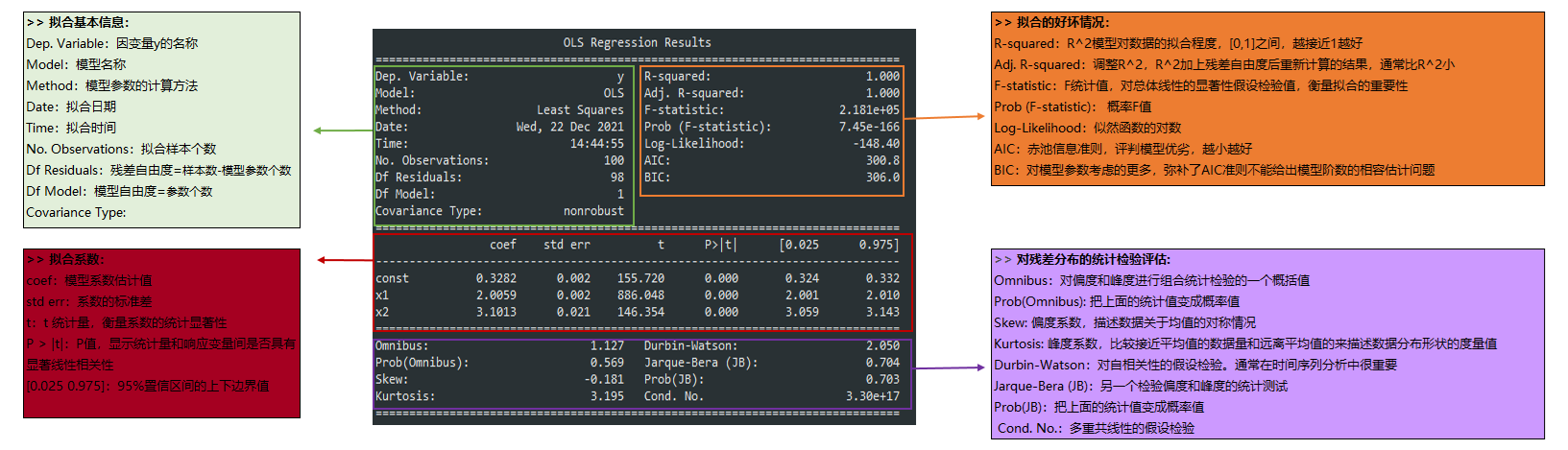##### Statsmodels 统计包之OLS回归